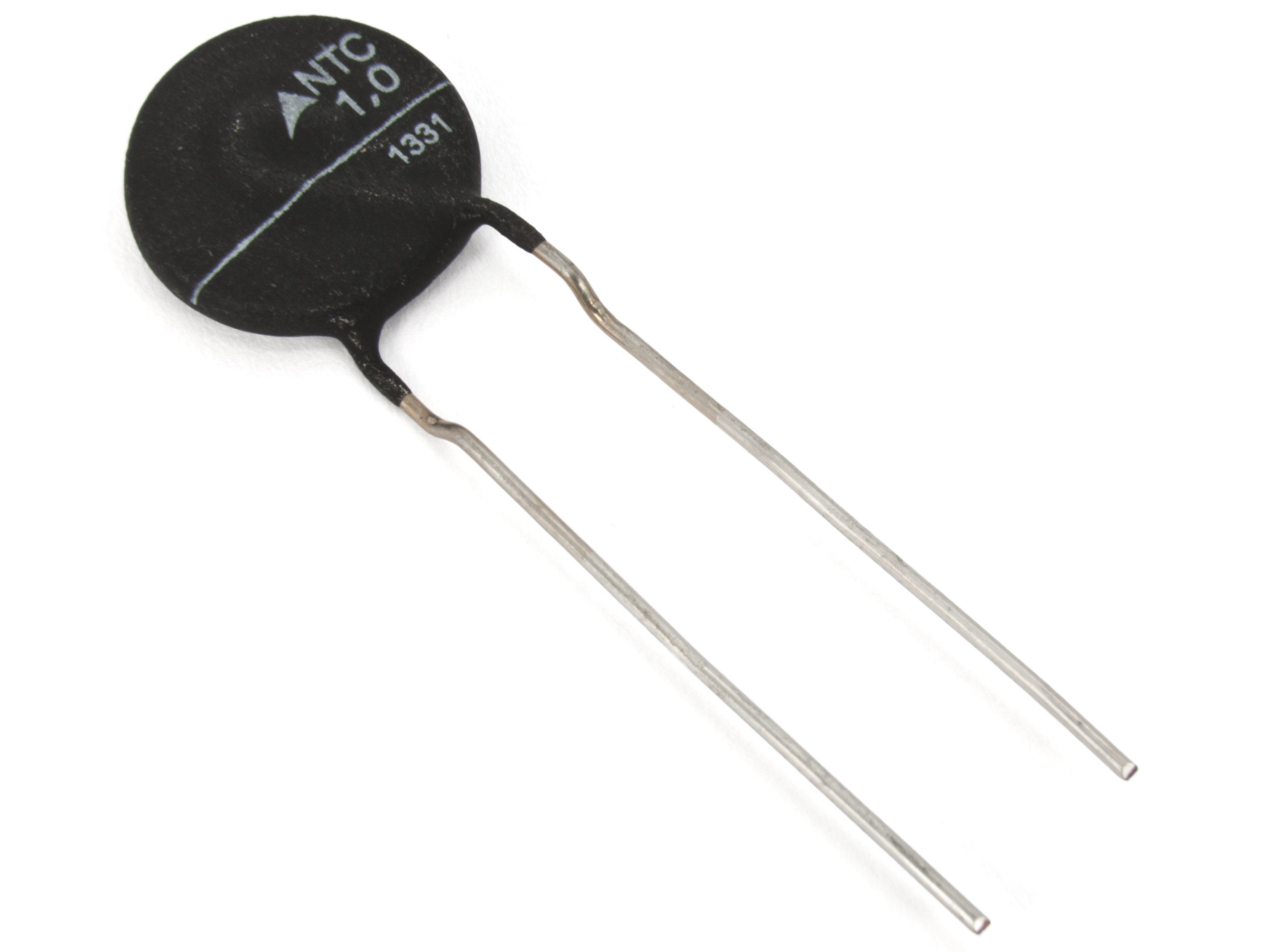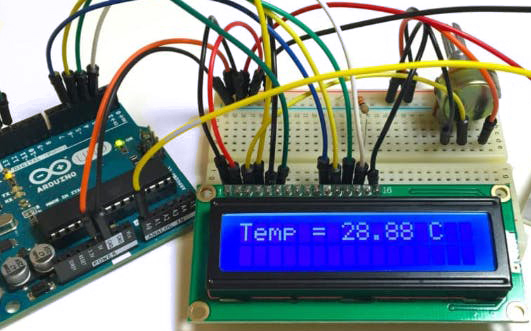It is an easy way to measure the exact temperature with a thermistor.

It is an easy way to measure the exact temperature with a thermistor.

Thermistor

The important segment in this circuit is Thermistor, which has been utilized to recognize the rise in temperature. The thermistor is temperature sensitive resistor, whose resistance modifies as per the temperature.

There are two different types of thermistor NTC and PTC, we have used as an NTC type thermistor. NTC thermistor is a resistor whose resistance diminishes as arise in temperature while in PTC it will improve the resistance as arise in temperature.Vout= (Vin * Rt) / (R + Rt)

Rt value will be:

Rt = R (Vin/Vout) – 1

Here, Rt will be the resistance of the thermistor and the value of R is 10k ohm.

Calculation of Temperature from the Thermistor resistance

T = 1 / (A + Bln(Rt) + Cln (Rt)3 )

Where, A, B and C are the constants,

So, for estimating the temperature we require the value of thermistor resistance only.```        ```#include <math.h>
#include "LiquidCrystal.h"
LiquidCrystal lcd(44,46,40,52,50,48);
float A = 1.009249522e-03, B = 2.378405444e-04, C = 2.019202697e-07;
float T,logRt,Tf,Tc;
float Thermistor(int Vo) {
logRt = log(10000.0*((1024.0/Vo-1)));
T = (1.0 / (A + B*logRt + C*logRt*logRt*logRt));  // We get the temperature value in Kelvin from this Stein-Hart equation
Tc = T - 273.15;                     // Convert Kelvin to Celcius
Tf = (Tc * 1.8) + 32.0;              // Convert Kelvin to Fahrenheit
return T;
}

void setup(){
lcd.begin(16,2);
lcd.clear();
}

void loop()
{
lcd.setCursor(0,0);
lcd.print("Temp:");
lcd.print("k ");

lcd.setCursor(0,1);
lcd.print((Tc));
lcd.print(" C ;");

lcd.setCursor(9,1);
lcd.print((Tf));
lcd.print(" F");
delay(800);
}```
```